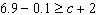Name:    Quiz 8.1-8.2

Multiple Choice
Identify the choice that best completes the statement or answers the question.

Write the word sentence as an inequality.

1.

A number k is less than 52.
 a.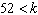c.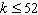b.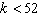d.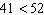2.

A number b is at least –16.
 a.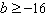c.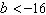b.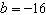d.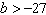Graph the inequality on a number line.

3.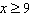a.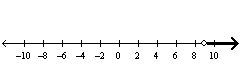c.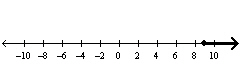b.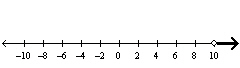d.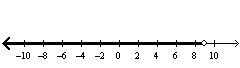4.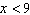a.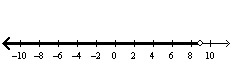c.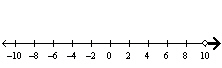b.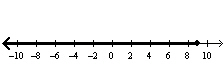d.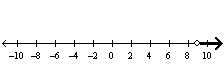5.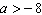a.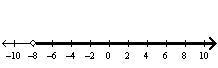c.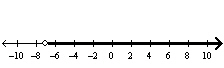b.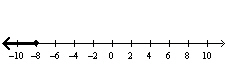d.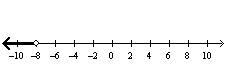6.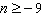a.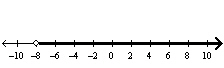c.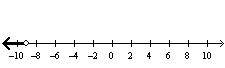b.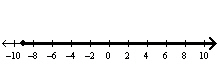d.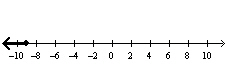7.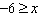a.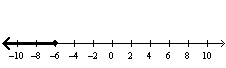c.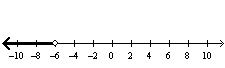b.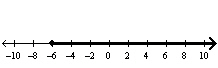d.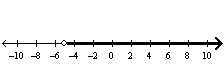Solve the inequality. Graph the solution.

8.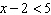a.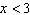;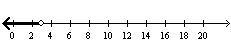c.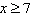;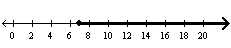b.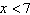;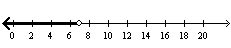d.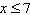;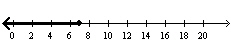9.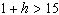a.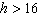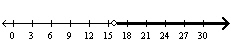c.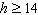;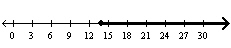b.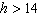;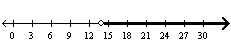d.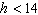;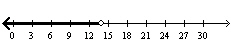10.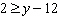a.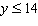;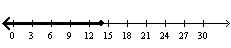c.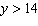;b.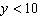;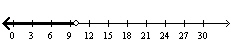d.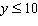;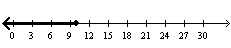11.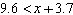a.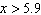;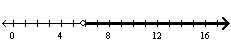c.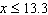;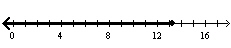b.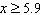;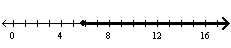d.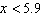;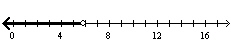12.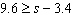a.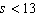;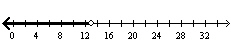c.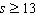;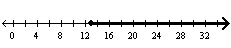b.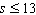;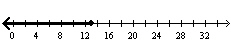d.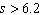;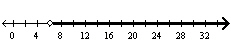13.

Your carry-on bag can weigh at most 40 pounds. Your bag weighs in at 25 pounds. Write and solve an inequality to represent how much more weight you can add to the bag and still meet the requirement.
 a.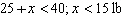c.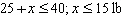b.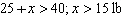d.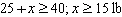Write the word sentence as an inequality. Then solve the inequality.

14.

Four less than a number is more than 15.
 a.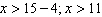c.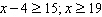b.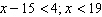d.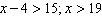Write an inequality and a word sentence that represent the graph.

1.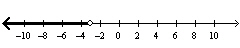2.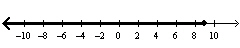Tell whether the statement is sometimes, always, or never true. Explain your reasoning.

3.

A number that is a solution of the inequality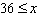is also a solution of the inequality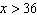.

4.

You are fishing in a river and are allowed to catch at most 3 striped bass. Each striped bass must be no less than 13 inches in length.
a. Write and graph an inequality to represent the number of striped bass you are allowed to catch.
b. Write and graph an inequality to represent the length of each striped bass you are allowed to catch.

Solve the inequality. Graph the solution.

5.